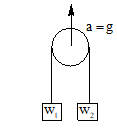Two weight W1 and  W2 are suspended as shown in figure. When the pulley is pulled up with an            acceleration g, then the tension in the string is

# Two weight W1 and  W2 are suspended as shown in figure. When the pulley is pulled up with an            acceleration g, then the tension in the string is1. A

$\frac{{\text{4w}}_{\text{1}}{\text{w}}_{\text{2}}}{{\text{w}}_{\text{1}}+{\text{\hspace{0.17em}w}}_{\text{2}}}$

2. B

$\frac{{\text{w}}_{\text{1}}{\text{w}}_{\text{2}}}{{\text{w}}_{\text{1}}+{\text{\hspace{0.17em}w}}_{\text{2}}}$

3. C

$\frac{{\text{2w}}_{\text{1}}{\text{w}}_{\text{2}}}{{\text{w}}_{\text{1}}+{\text{\hspace{0.17em}w}}_{\text{2}}}$

4. D

$\frac{{\text{w}}_{\text{1}}+{\text{w}}_{\text{2}}}{2}$

Register to Get Free Mock Test and Study Material

+91

Verify OTP Code (required)

### Solution:

$\text{T}=\frac{{\text{2m}}_{\text{1}}{\text{m}}_{\text{2}}}{{\text{m}}_{\text{1}}+{\text{m}}_{\text{2}}}\left(\text{g}+\text{a}\right)=\frac{4{W}_{1}{W}_{2}}{{W}_{1}+{W}_{2}}$Register to Get Free Mock Test and Study Material

+91

Verify OTP Code (required)